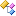﻿NReco.CF.Taste.Impl.Similarity Namespace
 NReco.CF.Taste.Impl.Similarity Namespace NReco.Recommender Class Library

Classes

ClassDescriptionAbstractItemSimilarityAbstractSimilarity
Abstract superclass encapsulating functionality that is common to most implementations in this package.AveragingPreferenceInferrer
Implementations of this interface compute an inferred preference for a user and an item that the user has not expressed any preference for. This might be an average of other preferences scores from that user, for example. This technique is sometimes called "default voting".CachingItemSimilarity
Caches the results from an underlying IItemSimilarity implementation.CachingUserSimilarity
Caches the results from an underlying IUserSimilarity implementation.CityBlockSimilarity
Implementation of City Block distance (also known as Manhattan distance) - the absolute value of the difference of each direction is summed. The resulting unbounded distance is then mapped between 0 and 1.EuclideanDistanceSimilarity

An implementation of a "similarity" based on the Euclidean "distance" between two users X and Y. Thinking of items as dimensions and preferences as points along those dimensions, a distance is computed using all items (dimensions) where both users have expressed a preference for that item. This is simply the square root of the sum of the squares of differences in position (preference) along each dimension.

The similarity could be computed as 1 / (1 + distance), so the resulting values are in the range (0,1]. This would weight against pairs that overlap in more dimensions, which should indicate more similarity, since more dimensions offer more opportunities to be farther apart. Actually, it is computed as sqrt(n) / (1 + distance), where n is the number of dimensions, in order to help correct for this. sqrt(n) is chosen since randomly-chosen points have a distance that grows as sqrt(n).

Note that this could cause a similarity to exceed 1; such values are capped at 1.

Note that the distance isn't normalized in any way; it's not valid to compare similarities computed from different domains (different rating scales, for example). Within one domain, normalizing doesn't matter much as it doesn't change ordering.GenericItemSimilarity
A "generic" IItemSimilarity which takes a static list of precomputed item similarities and bases its responses on that alone. The values may have been precomputed offline by another process, stored in a file, and then read and fed into an instance of this class.GenericItemSimilarity ItemItemSimilarityGenericUserSimilarityGenericUserSimilarity UserUserSimilarityLogLikelihoodSimilarity
Similarity test is based on the likelihood ratio, which expresses how many times more likely the data are under one model than the other.LongPairMatchPredicatePearsonCorrelationSimilarity
An implementation of the Pearson correlation.SpearmanCorrelationSimilarity
Like PearsonCorrelationSimilarity, but compares relative ranking of preference values instead of preference values themselves. That is, each user's preferences are sorted and then assign a rank as their preference value, with 1 being assigned to the least preferred item.TanimotoCoefficientSimilarity
An implementation of a "similarity" based on the Tanimoto coefficient, or extended Jaccard coefficient.UncenteredCosineSimilarity
An implementation of the cosine similarity. The result is the cosine of the angle formed between the two preference vectors.